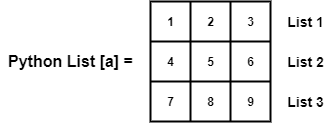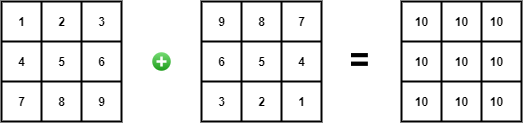# Python 3 Program To Add Two Matrices

1767

Here you will learn a simple Python 3 program to add two matrices. To understand this program, you should have the knowledge of following basic concepts of Python programming language:

• Lists
• For and nested for loops

If you are looking for some good books to learn Python, then do check out our recommended list.

## Python 3 Program To Add Two Matrices

We will use Python lists to store our matrices. We will cover lists in detail later but for now, let’s understand it from a top-level view. A Python list is basically an array but it can store homogeneous datatypes. For example, in a list, you can store strings, numbers, bool, or any combination of these data types.

In other simple words, the list is a collection of data that is ordered and changeable. Lists also support duplicate items.

Syntax of declaring Lists:

`myFriends = ["Himanshu", "Abhishek", "Vivek", 1, 2, 3]`

The following list myFriends now stores string values and integer values.

Likewise, you can also store multiple lists inside a list. Using this feature of Python lists, we can store our matrices.

To store a matrix of 3 by 3, we can create a list and define three lists with numbers, as shown in the diagram below.Therefore, to add two matrices, we simply have to create two lists (with three sub-lists of 3 numbers) and add them together. Please refer to the diagram below for a better understanding of the concept.To iterate through these lists, we can use for loops and add each number to its corresponding number in the second list and store the result in a third list.

### Source Code

```a = [[1, 2, 3],
[4, 5, 6],
[7, 8, 9]]
b = [[9, 8, 7],
[6, 5, 4],
[3, 2, 1]]
sum = [[0, 0, 0],
[0, 0, 0],
[0, 0, 0]]
for i in range(len(a)):
for j in range(len(a)):
sum[i][j] = a[i][j] + b[i][j]

for i in sum:
print(i)```

[10, 10, 10]

[10, 10, 10]

[10, 10, 10]

### Wrapping Up

In this tutorial, you learn how to add two matrices. Stay tuned for more Python 3 programs on working with matrices such as multiplication, subtraction, etc.

Let me know if there are any tutorials or concepts, you would like us to cover in our future posts.

Enjoy Python learning :)